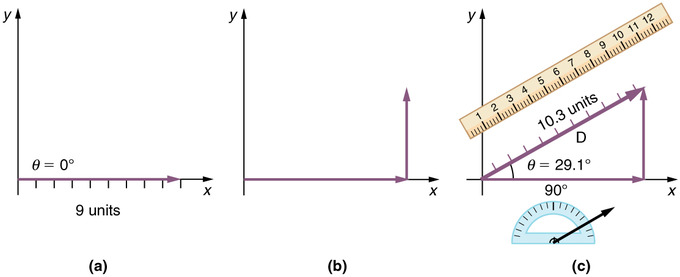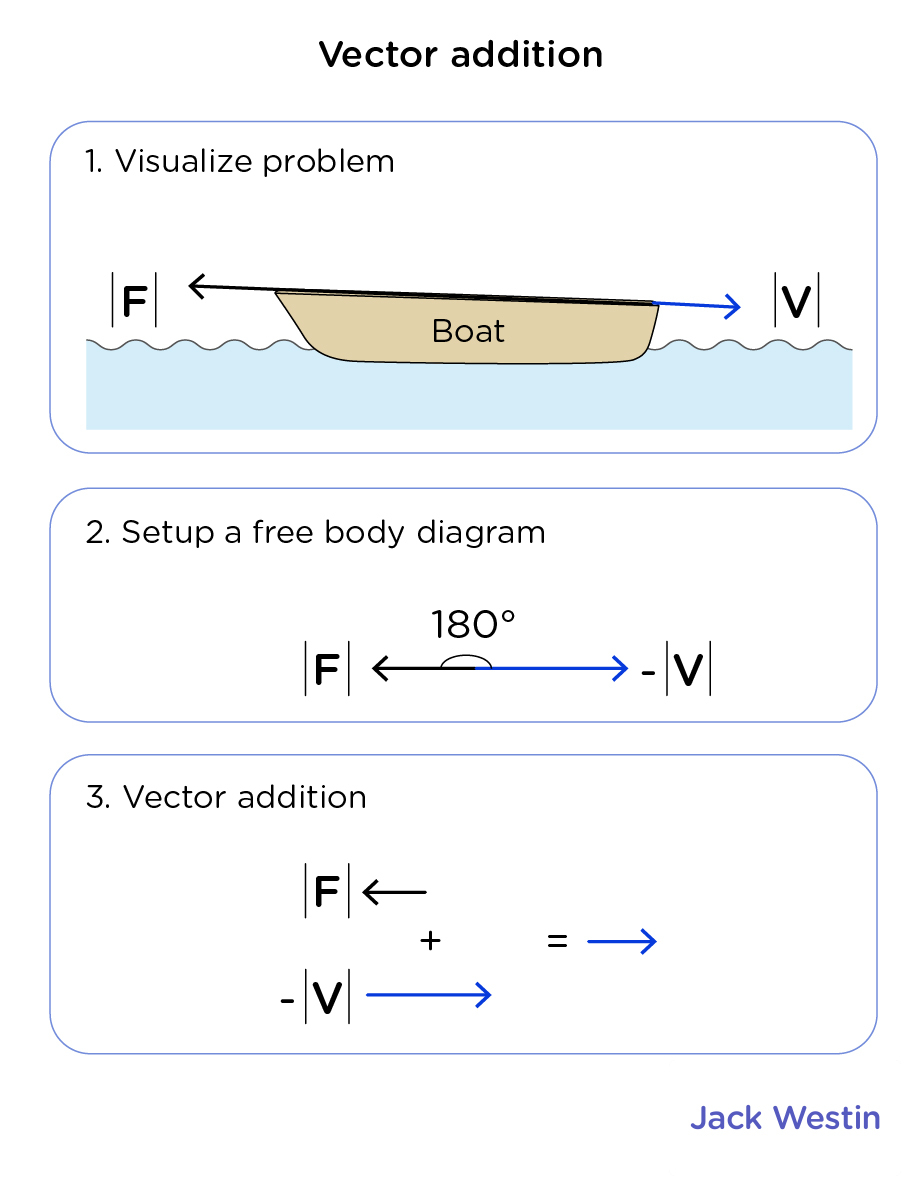Cyber Sale Extended 3 Days: ALL MCAT Products & Admissions Services

MCAT Content / Translational Motion / Vector Addition

Topic: Translational Motion

Vectors may be added or subtracted graphically by laying them end to end on a set of axes.

One of the ways in which representing physical quantities as vectors makes analysis easier is the ease with which vectors may be added to one another. Since vectors are graphical visualizations, addition and subtraction of vectors can be done graphically.

The graphical method of vector addition is also known as the head-to-tail method. To start, draw a set of coordinate axes. Next, draw out the first vector with its tail (base) at the origin of the coordinate axes. For vector addition it does not matter which vector you draw first since addition is commutative, but for subtraction ensure that the vector you draw first is the one you are subtracting from. The next step is to take the next vector and draw it such that its tail starts at the previous vector’s head (the arrow side). Continue to place each vector at the head of the preceding one until all the vectors you wish to add are joined together. Finally, draw a straight line from the origin to the head of the final vector in the chain. This new line is the vector result of adding those vectors together.Graphical Addition of Vectors: The head-to-tail method of vector addition requires that you lay out the first vector along a set of coordinate axes. Next, place the tail of the next vector on the head of the first one. Draw a new vector from the origin to the head of the last vector. This new vector is the sum of the original two.

To subtract vectors the method is similar. Make sure that the first vector you draw is the one to be subtracted from. Then, to subtract a vector, proceed as if adding the opposite of that vector. In other words, flip the vector to be subtracted across the axes and then join it tail to head as if adding. To flip the vector, simply put its head where its tail was and its tail where its head was.

Remember:

• The vector sum of all components of a vector equal to the vector itself.
• An operation involving a vector and a vector may or may not result in a vector (kinetic energy from the square of vector velocity results in scalar energy).
• An operation involving a vector and a scalar always results in a vector.
• An operation involving a scalar and a scalar always results in a scalar.Practice Questions

Swine flu in Finland

MCAT Official Prep (AAMC)

Physics Question Pack Question 116

Practice Exam 4 C/P Section Question 12

Key Points

• To add vectors, lay the first one on a set of axes with its tail at the origin. Place the next vector with its tail at the previous vector’s head. When there are no more vectors, draw a straight line from the origin to the head of the last vector. This line is the sum of the vectors.

• To subtract vectors, proceed as if adding the two vectors, but flip the vector to be subtracted across the axes and then join it tail to head as if adding.

• Adding or subtracting any number of vectors yields a resultant vector.

• The vector sum of all components of a vector equal to the vector itself.

• An operation involving a vector and a vector may or may not result in a vector (kinetic energy from the square of vector velocity results in scalar energy)

• An operation involving a vector and a scalar always results in a vector.

• An operation involving a scalar and a scalar always results in a scalar.

Key Terms

Origin: The center of a coordinate axis, defined as being the coordinate 0 in all axes.

Coordinate axes: A set of perpendicular lines which define coordinates relative to an origin. Example: x and y coordinate axes define horizontal and vertical position.

Billing Information